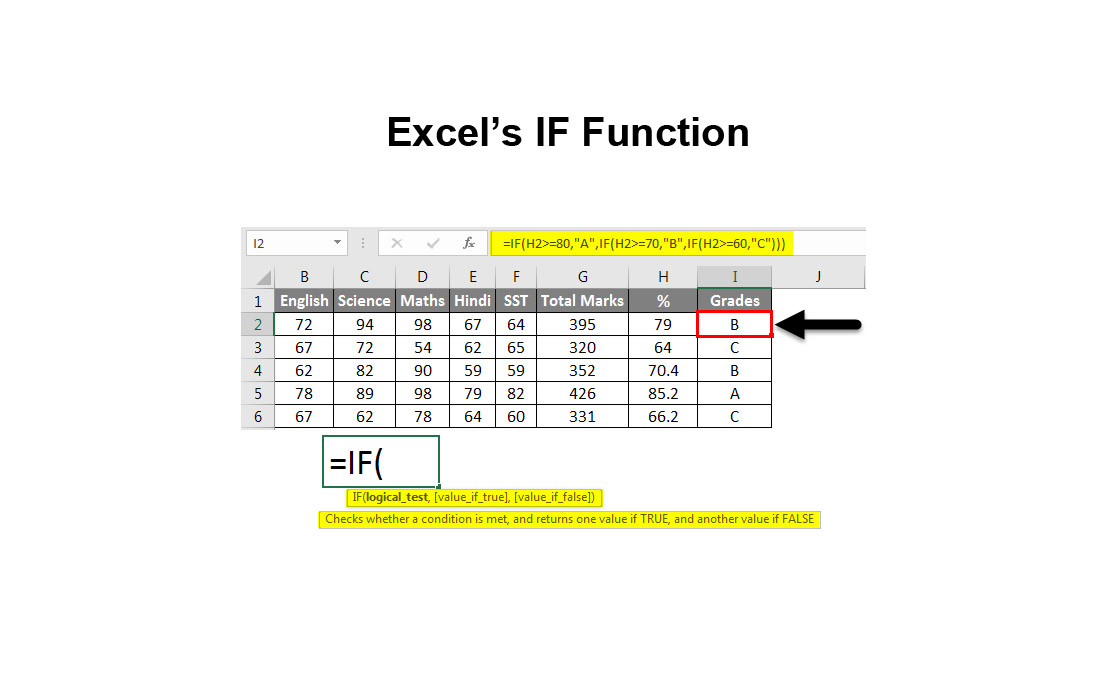# Excel’s IF FunctionIn Excel the IF function is used to return a condition based on both the TRUE and FALSE evaluations of an argument.

The syntax for the IF function is:

=IF ( logical_test, value_if_true, value_if_false )

logical_test – a value or expression that is tested to see if it is true or false.
value_if_true – the value that is displayed if logical_test is true.
value_if_false – the value that is displayed if logical_test is false.

Before you get into creating an IF function, it is best to write out exactly what you are attempting to accomplish.  If you can explain it logically, you can write the arguments!

If cell A1 is equal to or greater than “20”, then return the value “Approved”; otherwise, return the value “Denied”

Your brain is already aware of steps to take and how to proceed by the reading the statement above.  Excel is half way there as well!  Let’s take the function apart and identify the arguments:

=IF( (notifies Excel we are going to be starting an IF function)
A1 (the cell we are wanting to verify)
>=20, (asking if the value is equal to or greater than “20”
“Approved”, (what to return if the above query is TRUE
“Denied”) (what to return if the above query is FALSE

Putting this all together, we will come up with the following string:

=IF(A1>=20, “Approved”, “Denied”)

Now that we have the arguments settled, we can test it out.  By inserting the value “10” in  cell A1, we will be given the value “Denied” in the cell where this function is located.

By inserting the value “22” in cell A1, we will be given the value “Approved” in the cell where this function is located.

Now what if you wanted to perform another function in the event that the above value was returned as FALSE (“Denied”)?  We can evaluate the need to nest the IF functions:

If cell A1 is equal to or greater than “20”, then return the value “Approved”; otherwise, if cell B1+A1 is equal to or greater than “40”, then return the value  “Approved”; otherwise, return the value “Denied”

Here, we have added another set of arguments to our previous scenario.  By adding another IF function, we are redirecting the FALSE argument to perform another process before ending.  Adding to the code above, we would now write:

=IF(A1>=20, “Approved”, IF((A1+B1)>=40, “Approved”, “Denied”))